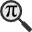## General

Display information for equation id:math.262235.2 on revision:262235

* Page found: Conical combination (eq math.262235.2)

(force rerendering)

Cannot find the equation data in the database. Fetching from revision text.

Occurrences on the following pages:

Hash: 1df107efd8d34de0c4d18ba3eafe211c

TeX (original user input):

\alpha_i\,


TeX (checked):

\alpha _{i}\,


### LaTeXML (experimental; uses MathML) rendering

MathML (950 B / 377 B) :${\displaystyle\alpha_{i}\,}$
<math xmlns="http://www.w3.org/1998/Math/MathML" id="p1.1.m1.1" class="ltx_Math" alttext="{\displaystyle\alpha_{i}\,}" display="inline">
<semantics id="p1.1.m1.1a">
<msub id="p1.1.m1.1.4a" xref="p1.1.m1.1.4.cmml">
<mi id="p1.1.m1.1.1" xref="p1.1.m1.1.1.cmml">α</mi>
<mi id="p1.1.m1.1.2.1" xref="p1.1.m1.1.2.1.cmml">i</mi>
</msub>
<annotation-xml encoding="MathML-Content" id="p1.1.m1.1b">
<apply id="p1.1.m1.1.4.cmml" xref="p1.1.m1.1.4">
<csymbol cd="ambiguous" id="p1.1.m1.1.4.1.cmml" xref="p1.1.m1.1.4">subscript</csymbol>
<ci id="p1.1.m1.1.1.cmml" xref="p1.1.m1.1.1">𝛼</ci>
<ci id="p1.1.m1.1.2.1.cmml" xref="p1.1.m1.1.2.1">𝑖</ci>
</apply>
</annotation-xml>
<annotation encoding="application/x-tex" id="p1.1.m1.1c">{\displaystyle\alpha_{i}\,}</annotation>
</semantics>
[/itex]


SVG (1.945 KB / 1019 B) :

### MathML with SVG or PNG fallback (recommended for modern browsers and accessibility tools) rendering

SVG image empty. Force Re-Rendering

SVG (0 B / 8 B) :

PNG (0 B / 8 B) :

$\alpha _{i}\,$## Translations to Computer Algebra Systems

### Translation to Maple

In Maple: alpha[i]

I: You use a typical letter for a constant [the imaginary unit == the principal square root of -1].

We keep it like it is! But you should know that Maple uses I for this constant.

If you want to translate it as a constant, use the corresponding DLMF macro \iunit

\alpha: Could be the second Feigenbaum constant.

But this system don't know how to translate it as a constant. It was translated as a general letter.


i: the imaginary unit == the principal square root of -1 was translated to: i

### Translation to Mathematica

In Mathematica: Subscript[\[Alpha], i]

I: You use a typical letter for a constant [the imaginary unit == the principal square root of -1].

We keep it like it is! But you should know that Mathematica uses I for this constant.

If you want to translate it as a constant, use the corresponding DLMF macro \iunit

\alpha: Could be the second Feigenbaum constant.

But this system don't know how to translate it as a constant. It was translated as a general letter.


i: the imaginary unit == the principal square root of -1 was translated to: i

## Similar pages

Calculated based on the variables occurring on the entire Conical combination page

## Identifiers

• $\alpha _{i}$### MathML observations

0results

0results

no statistics present please run the maintenance script ExtractFeatures.php

0 results

0 results